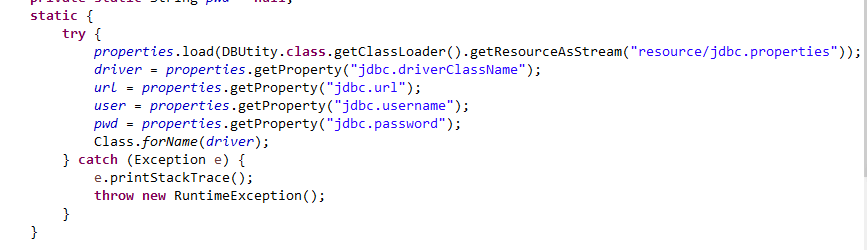``````public TestUtil() {
。。。
}

``````

2个回答`````` static {
try{
// 初始化log4j
String log4jPath = "";
if(Constant.LOG_MODE == 1){
// 配置线上地址
logger.info("Log4j线上生产模式初始化。。。");
}else{
// 配置本地地址
logger.info("Log4j线下开发模式初始化。。。");
}
logger.info("初始化Log4j。。。。");
logger.info("path is "+ log4jPath);
PropertyConfigurator.configure(log4jPath);
}catch (Exception e){
logger.error(e.toString());
}
}
``````package complex_number; import java.util.*; public class ComplexNumber { public static void main(String[] args){ java.util.Scanner scanner = new java.util.Scanner(System.in); float m_dRealPart; float m_dImaginPart; System.out.println("Please enter the real and imaginary parts of the plural："); m_dRealPart=scanner.nextFloat(); m_dImaginPart=scanner.nextFloat(); System.out.println("\n"); System.out.println("What kind of operation do you want to do? (1 Plus, 2 minus, 3 multiply, 4 divide)"); } /*构造函数，ComplexNumber,将实部和虚部置0*/ public ComplexNumber(){ m_dRealPart=0; m_dImaginPart=0; } 请问在构造函数的时候为什么会错？

C++ 默认构造函数问题

c++ 派生类 拷贝构造函数 调用基类的构造函数

int lastnum = getNum(source.getProperty("fileName")); public static int getNum(String Filename) throws IOException { InputStream myxls; myxls = new FileInputStream(Filename); sr = new XSSFWorkbook(myxls); XSSFSheet sheet = sr.getSheetAt(0);// 第一个工作表 return sheet.getLastRowNum();// 获取第一个工作表的行数 } 第一行报错：缺省构造函数不能处理隐式超构造函数抛出的异常类型 IOException。必须定义显式构造函数

class MyClass{ int value; public: MyClass(int n):value(n){} int getValue()const{return value;} }; 则类MyClass的构造函数的个数是______。 A、1个 B、2个 C、3个 D、4个 我认为答案是A，但是很多书答案都是B，说是还有一个默认构造函数。已经定义了构造，怎么还会有默认构造，难道是默认复制？本人小白，求指教

C++显式调用构造函数初始化对象有用到拷贝构造函数么？

C++语言的构造函数里能不能跳出构造函数，直接返回null

C++语言的构造函数里能不能跳出构造函数，直接返回null，比如当参数检查不符合要求的时候都是怎么做的？

C++中派生类的构造函数一定得调用基类的构造函数吗

c++中new是怎么调用构造函数的

c++中new是怎么调用构造函数的 c++中new是怎么调用构造函数的

c++函数调用时拷贝构造函数和析构函数

C++对象数组赋值调用构造函数问题

C++类的默认构造函数问题

``` #include<iostream> #include<string> using namespace std; class A { public : int val; //这里把A的默认构造函数屏蔽 //A(){cout<<"A()"<<endl;val=-1;} A(int n){cout<<"A(int)"<<endl;val=n;} }; class AA { public: A a ; //因为A不存在默认构造函数，所以编译器也就无法为AA生成默认构造函数 //AA(){ ;} }; int main() { AA aa; } ``` 上面的代码，把class A的无参构造函数屏蔽掉，编译器就无法为A提供默认构造函数， 在AA类中定义一个A a，AA也将无法默认初始化，所以程序编译的时候会报错，现在想在不修改A的情况下，对AA和main（）作出一些修改，让程序编译通过，想问问老哥们有没有解决的办法。 如果不能解决的话是不是就是说，这样的定义的无默认初始化的class A无法在别的类中实例化（c++98编译器）？

JAVA 怎么限制类型必须有公共无参构造函数?

QLibray 动态加载库时,怎么调用动态库中类的构造函数

#include<iostream> using namespace std; class IntClass { private: int value; public: //转换int的转换构造函数 IntClass(int intValue) { value = intValue; } IntClass(IntClass &p) { } int getValue() const { return value; } }; int main() { IntClass a=1; a=2; } 如果去掉复制构造函数报错就消失了，但是a(1)这样然后下面写a=2就没问题。还有就是 #include<iostream> using namespace std; //点类 class Point{ public : Point(int xx,int yy=0); // Point(Point &p); Point(){ x=4; y=4; } int getX(){ return x; } int getY(){ return y; } private : int x,y; }; Point::Point(int xx,int yy) { x=xx; y=yy; } //Point::Point(Point &p) //{ // x=p.x; // y=p.y; //} //线类 class Line{ public : Line(Point xp1,Point xp2); Line(Line &m); double getlen(){ return len; } private : double len; Point p1,p2; }; Line::Line(Point xp1,Point xp2):p1(xp1),p2(xp2) // { cout<<xp1.getX()<<ends<<xp2.getX()<<ends<<p1.getX()<<ends<<p2.getX()<<endl; len=3; } Line::Line(Line &m):p1(m.p1),p2(m.p2) { len=m.len; cout<<len; } int main() { Point myp1(1,1),myp2(4,5); Line line(myp1,myp2); Line line2(line); myp1=Point(1,4); myp1=1; cout<<myp1.getY(); cout << Line(Point(1), Point(4)).getlen() << endl; } 像这样如果注释掉复制构造函数就没关系不报错但是恢复又会报错，请问这是问什么

c++中子类对象创建时，如果父类包含有有参构造函数,则必须在子类构造函数的初始化列表中 初始化，可是为什么必须要这样做，我知道是为了初始化子类继承父类的成员变量。java中是在子类构造函数的 第一行显示调用父类有参构造初始化，我想问的是c++必须在子类构造函数的初始化列表中进行 初始化仅仅是语法的规定么。比如:c++也可以在子类构造函数第一行调用父类构造函数初始化啊 ，有没有其它更好的解释

Java基础知识面试题（2020最新版）

Intellij IDEA 实用插件安利

1. 前言从2020 年 JVM 生态报告解读 可以看出Intellij IDEA 目前已经稳坐 Java IDE 头把交椅。而且统计得出付费用户已经超过了八成（国外统计）。IDEA 的...

MySQL数据库面试题（2020最新版）

2020阿里全球数学大赛：3万名高手、4道题、2天2夜未交卷

HashMap底层实现原理，红黑树，B+树，B树的结构原理 Spring的AOP和IOC是什么？它们常见的使用场景有哪些？Spring事务，事务的属性，传播行为，数据库隔离级别 Spring和SpringMVC，MyBatis以及SpringBoot的注解分别有哪些？SpringMVC的工作原理，SpringBoot框架的优点，MyBatis框架的优点 SpringCould组件有哪些，他们...

Python爬虫，高清美图我全都要（彼岸桌面壁纸）

Vue回炉重造之router路由（更新中）

Java岗开发3年，公司临时抽查算法，离职后这几题我记一辈子